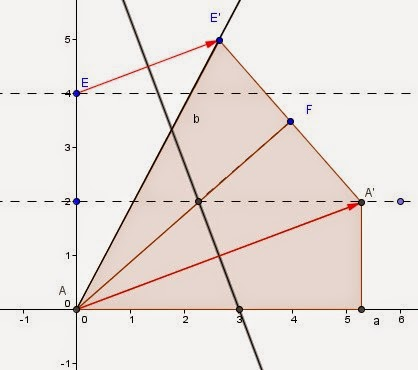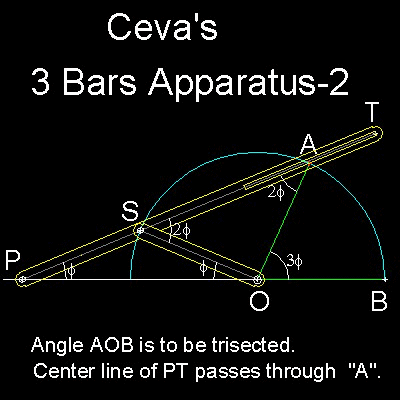## Sunday, 10 February 2019

### Trisecting the General Angle, A Plethora of Pretty Approaches

I recently wrote about a comparison between the approaches to trisecting an angle by Viete and the method used by Jost Burgi as presented in Pitiscus' famous Trigonometria. In response I received several (admittedly very kind in tone)  tweets explaining to me that it "couldn't be done".  This misunderstanding is so pervasive that two of the comments included statements that they didn't read the blog, but thought they should tell me that is was "well known" that you "just can't do it."
Well, for an impossible task, mathematicians of the highest order have been trisecting the general angle for at least 2000 years.  I have gone on record before as saying that I thought this would be a nice topic for almost any level of high school student since the methods of doing it touch on almost any level of mathematical ability.

So without resorting to "Oh, Yeah?" or "So's your mama" I would like to point out several approaches that I think might be appropriate for introduction to high school level students.

I begin with the method of the neusis, a straight edge with a segment marked on it that was used by Viete in the previously mentioned blog.

The Neusis Approach of Viete (and Archimedes

The relation of trisecting to the cubic equation $x^3 - 3x = 2a)\ was well known, and Viete knew that with a suitable change of variables, it could be used to solve any cubic with all real roots.The method he would use dates back to the early Greeks, and was known and used by Archimedes. I have tried to illustrate the "neusis" approach with static pictures from a very dynamic program, but teachers who are interested in making this clearer to their students can easily construct an interactive model for their students. We begin with an isosceles triangle with a base angle at the origin and the angle to be trisected and the vertex on a circle centered at the origin. The marked ruler is represented her by the ray EG, and for our purpose has G fixed on the circle passing through the top vertex of the triangle. In use, the mathematician would lay his straightedge along one of the two congruent sides of the triangle, and mark EG so that it was the length of a side. Then holding E on the x-axis, and G on the circle, he would move the points along the respective curves until the straight edge passed through the vertex angle at C . The resulting at \(\angle$ AEG is the trisector.

For ease in seeing a simple proof, I add one drawing with a second isosceles triangle shown:

There are actually three isosceles triangles in the figure, the two shaded ones, $\Delta$ACH , and $\Delta$AGE and the one between them $\Delta$CAG.   If we label the $\angle$ CAH to be trisected as ythen it's supplementary angle, $\angle$ CAE is 180 - y.  Now if we call the equal angles $\angle$AEG and $\angle$GAE each x, then the exterior angle $\angle$AGC to this isosceles triangle must be 2x, as must it's base angle partner $\angle$ACG.  This leaves $\angle$ CAG as 180 - 4x.  Adding back the value x at $\angle$GAE means that $\angle$CAE is equal to 180 - 3x, but we already know it is equal to 180-y, so y=3x as we sought.

(I think, technically, all the methods that use fixed curves are also of the neusis type, with the curve serving the role of the marked straight edge as the neusis.)

Trisecting an angle by Origami

You don't have to be able to make beautiful cranes or jumping frogs with folded paper to be able to trisect an angle.  This method works exactly the same as the carpenters square below, so I will take a little time to explain it.  This approach is usually credited to Hisashi Abe who did around 1980

If you take a standard sheet of paper, and draw an angle from the bottom left corner into the page so that the bottom of the page is one ray of the angle (otherwise you can draw it anywhere and then cut the  sheet along one ray of the triangle... oh just do it the easy way).
Now pick a point along the perpendicular to the base, and make a fold. Almost any point will do, but leave some room on your paper for another fold of the same size, and a little above that for the 'folding' part of the method.

At this point we make a second fold equal to the first. and we are ready to do the impossible, trisect the angle.

Fold the corner (A) so that it lies along the first fold while making the edge of the second fold (E) falls onto the side of the triangle, AC.  The Ray AC is the bisector of the original angle.

The proof is in three similar right triangles that result from the folds, shown below.  All three of the triangles have a short leg that is the same length as the distance between our folds, and one pair share a common hypotenuse, while the other share a common leg, so all three are congruent, and thus we have three copies of an angle that is 1/3 of the original angle.Trisecting by the use of special curves
There are a number of curves that have been used as an aide in the trisection of an angle.  At the time I checked, the Wikipedia article on Trisectrix listed nine:
I will limit myself to the first and the last. My only justification is that the first is such a novel and attractive approach, and the last is so appropriate for upper algebra and precalculus classes due to graphic approach.

The Limacon trisection

The Limacon (which I am told is from the Latin word for snail) which has the equation  r=a (1+2 cos $\theta$ has the intersection of the inner loop at the origin. (The same image is translated so that the tip of the loop is at the origin by the equation r= 2a cos( $\frac{\theta}{3}$ )
If the angle we desire to trisect is drawn with the vertex at the tip of the inner loop and one ray along the positive x-axis, the other ray will pass through the limacon at some point P.   If we draw a segment from the origin  to the point P, the angle formed at P is the trisecting angle.image fom http://xahlee

To see why this is so, we need to add the generating circle for the limacon.  A circle centered at (1,0) with a radius of one. This one is from a Wolfram demonstration on Youtube by Izidor Hafner.
Once more the secret is revealed by isosceles triangles.  Note that $\Delta$ OSP in this figure and $\Delta$ SPA are both isosceles.  The  $\angle$ OSA is 180o - $\alpha$. Letting  $\angle$ SAP =  $\angle$ PSA both be x, then the exterior angle at $\angle$ OPS is 2x, as is its base pair $\angle$ SOP. This leads again to the fact that $\angle$  OSP is 180o- 4x, and if we add back $\angle$ PSA we are left with $\angle \alpha$ = 180o-3x = 180o - $\alpha$, as in the example with the neusis.

The Carpenter's Square or Gnomen
I have seen this approach dated as early as the Romans, but can find no evidence to support that.  If you are aware of such, I would love to be informed.
The Carpenter's square approach produces the same layout as the Origami method.
A nice illustration of the approach is given in A Mathematical Pandora's Box By Brian Bolt. In fact it is so nice, I have used it here. Show your appreciation to Mr Bolt by buying his puzzle book at the link below.First the width of the square is used to create a line parallel to one side of the angle to be trisected. Then the square is positioned so that the top side of the square falls along the vertex of the angle, with the corner of the square on one side of the angle, and the four inch mark on the leg of the square touching the previously created line.  (The standard square is 2" in width, so this makes the distance from the inner edge of the square to the constructed line the same as the width of the square.  As shown in the illustration, this creates the same three right triangles that made the paper folding method work, and a point at P, or a point at the 4" mark will serve to trisect the angle.There is a special trisecting tool called the tomahawk that is a variation of the Carpenter's square.  It seems to have been created in the first half of the 19th century.  Wikipedia says, "The inventor of the tomahawk is unknown, but the earliest references to it come from 19th-century France. It dates back at least as far as 1835, when it appeared in a book by Claude Lucien Bergery, Géométrie appliquée a l'industrie, a l'usage des artistes et des ouvriers (3rd edition).

The image (from Wolfram Mathworld) shows the design and it's use.  The semicircle on the bottom of the top edge RSTU serves the same purpose as the initial line drawn in the use of the carpenter's square, it insures the perpendicular distance TD will be the same as RS and TU for the three isosceles right triangles.Takaya Iwamoto
A mechanical approach using linkages was created by Giovanni Ceva in the middle of the 17th Century.  Ceva is known most (where he is known at all) for his theorem on concurrency of lines from the three vertices of a triangle, but he also worked in hydraulics and mechanics.  The method produces the same result as the neusis of Archimedes , using a three bar configuration. You may think of the bar PT as the straight edge of that method, and PS to be the marked distance.  .
Point O is place at the vertex of the angle to be trisected, with P on the extended base of the angle, and A is moved so that the bar PT pivots until A aligns with the second side of the angle.  This results, by the same isosceles triangles as before, in thTPB as the 1/3 angle.

I think the diversity of the many ways that have been used over time suggest the depth of the mathematical interest they create.  I imagine that these would be very interesting to high school age students, and if you should try them in a classroom I would love to know the response.